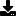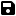Don't have an account yet? Register

# 100% real graphics, pure code, no shapes / images. Make 3D lines, fractal styles, tubes, spirals. M

by mJ (1 Submission)
Category: Graphics
Compatability: Visual Basic 5.0
Difficulty: Unknown Difficulty
Originally Published: Fri 4th January 2002
Date Added: Mon 8th February 2021
Rating:(1 Votes)

100% real graphics, pure code, no shapes / images. Make 3D lines, fractal styles, tubes, spirals. Mathematic & graphical example.

Rate 100% real graphics, pure code, no shapes / images. Make 3D lines, fractal styles, tubes, spirals. M

`''' This is just something I made to demonstrate''' what you can accomplish with PURE CODE.''' No shapes, no jpegs, just old school style coding.''' This DOESN'T look good at all, but you can make it better''' I'm sure, since I really am a newbie.'''''' Note the fractal type shapes, tubes,''' vector style lines, SMOOTH COLOR FILLS''' no flicker except during some really fast''' color cyclings.''' Also note some of the 3D-ish things you can do.'''''' This example will probably work on all''' versions of VB, but no promises.''' This was written in VB4 32bit.''' ''''''  -mJ  april 01 2002 ''''(())(())(())(())(())(())(())(())(())(())(())((xxx))'(())(())(())(())(())(())(())(())(())(())(())((eof))'''''''''''''''''''''''''''''''''''''''''''''''''''''''''''' You need to:'''''''' '''''''' 1) Make sure your form is named FORM1 [it is by default]'''''''' 2) Place this code into a command button ''''''''    or where ever you want to activate it from.Form1.BackColor = 0Form1.ForeColor = 10000For n = 1 To 4000 Step 5For x = 1 To 4000 Step 10Form1.ForeColor = Form1.ForeColor + 1Line (n, x)-(x, n)Next xNext n'''''''''''''''''''''''''''''''''''''''''''''''''''' next part ''''''''''''''''''''''''''''''''''''''''''''''''''''                     For n = 1 To 10 Step 2     For i = 1 To 6000     Form1.ForeColor = Form1.ForeColor + 1          Circle (i * 8.53, i * 3.58), i * 0.22Next i     Form1.ForeColor = Form1.ForeColor + 1          Line (i * 8.53, i * 3.58)-(n * 3.58, i * n)                           Next n'''''''''''''''''''''''''''''''''''''''''''''''''''' next part ''''''''''''''''''''''''''''''''''''''''''''''''''''  Form1.ForeColor = 22000  For c = 1 To 100 Step 5: Form1.ForeColor = Form1.ForeColor + 1  For b = 1 To 1000 Step 32: Form1.ForeColor = Form1.ForeColor + 1  For a = 1 To 1000 Step 64: Form1.ForeColor = Form1.ForeColor + 1  Circle (b * Sin(32) + 32, b * Cos(106) + 64), b  Circle (b * Sin(64), b * Cos(2)), b  Circle (b * Sin(64), b * Cos(64)), b  Next a: Form1.ForeColor = Form1.ForeColor + 1  Next b: Form1.ForeColor = Form1.ForeColor + 1  Next c: Form1.ForeColor = Form1.ForeColor + 1 '''''''''''''''''''''''''''''''''''''''''''''''''''' next part ''''''''''''''''''''''''''''''''''''''''''''''''''''Form1.ForeColor = 3.2 * RndFor n = 1 To 300 Step 3For x = 1 To 100 Step 5For i = 1 To 300 Step 4If Form1.ForeColor >= 16842715 Then Form1.ForeColor = 3.2 * RndForm1.ForeColor = Form1.ForeColor + xCircle (n * Sin(x) * 3.58, x * Cos(n) * 3.58), iNext iNext xNext n'''''''''''''''''''''''''''''''''''''''''''''''''''' next part ''''''''''''''''''''''''''''''''''''''''''''''''''''Form1.ForeColor = 3.2 * RndFor n = 1 To 400 Step 10For x = 1 To 500 Step 10For i = 1 To 400 Step 10If Form1.ForeColor <= 16842715 Then Form1.ForeColor = 3.2 / RndForm1.ForeColor = Form1.ForeColor + xCircle (n * Sin(x * 5) * 3.58, x * Cos(n * 5) * 3.58), iNext iNext xNext n'''''''''''''''''''''''''''''''''''''''''''''''''''' last part ''''''''''''''''''''''''''''''''''''''''''''''''''''For n = 1 To 1000 Step 5For x = 1 To 1000 Step 5Form1.ForeColor = Form1.ForeColor + 2Line (n, x)-(x, n)Circle (n, x), n + xNext xNext nEnd Sub`Download this snippetAdd to My Saved Code

# 100% real graphics, pure code, no shapes / images. Make 3D lines, fractal styles, tubes, spirals. M Comments

No comments have been posted about 100% real graphics, pure code, no shapes / images. Make 3D lines, fractal styles, tubes, spirals. M. Why not be the first to post a comment about 100% real graphics, pure code, no shapes / images. Make 3D lines, fractal styles, tubes, spirals. M.